×#### Thank you for registering.

One of our academic counsellors will contact you within 1 working day.

Click to Chat

1800-1023-196

+91-120-4616500

CART 0

• 0

MY CART (5)

Use Coupon: CART20 and get 20% off on all online Study Material

ITEM
DETAILS
MRP
DISCOUNT
FINAL PRICE
Total Price: Rs.

There are no items in this cart.
Continue Shopping• Complete JEE Main/Advanced Course and Test Series
• OFFERED PRICE: Rs. 15,900
• View Details

```Chapter 18: Surface Area and Volume of a Cuboid and Cube Exercise – 18.1

Question: 1

Find the lateral surface area and total surface area of a cuboid of length 80 cm, breadth 40 cm and height 20 cm.

Solution:

Given that:

Cuboid length (l) = 80 cm

Height (h) = 20 cm

We know that,

Total Surface Area = 2[lb + bh + hl]

= 2[(80)(40) + (40)(20) + (20)(80)]

= 2[3200 + 800 + 1600]

= 2

= 11200 cm2

Lateral Surface Area = 2[l + b]h

= 2[80 + 40]20

= 40

= 4800 cm2

Question: 2

Find the lateral surface area and total surface area of a cube of edge 10 cm.

Solution:

Cube of edge (a) = 10 cm

We know that,

Cube Lateral Surface Area = 4a2

= 4(10*10)

= 400 cm2

Total Surface Area = 6 a2

= 6 * 102

= 600 cm2

Question: 3

Find the ratio of the total surface area and lateral surface area of a cube.

Solution:

Total Surface Area of the Cube (TSA) = 6 a2

Where, a = edge of the cube

And, Lateral surface area of the Cube (LSA) = 4 a2

Where, a = edge of the cube

Hence, Ratio of TSA and LSA = 6a2/4a2 = 3/2 is 3:2.

Question: 4

Mary wants to decorate her Christmas tree. She wants to place the tree on a wooden block covered with colored paper with a picture of Santa Claus on it. She must know the exact quantity of paper to buy for this purpose. If the box has length, breadth, and height as 80 cm, 40 cm and 20 cm respectively. How many square sheets of paper of side 40 cm would she require?

Solution:

Given that:

Mary wants to paste a paper on the outer surface of the wooden block. The quantity of the paper required would be equal to the surface area of the box which is of the shape of a cuboid.

The dimensions of the wooden block are:

Length (l) = 80 cm

Height (h) = 20 cm

Surface Area of the wooden box = 2[lb + bh + hl]

= 2[(80*40) + (40*20) + (20*80)]

= 2

= 11200 cm2

The Area of each sheet of the paper = 40 x 40 cm2

= 1600 cm2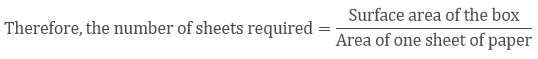=11200/1600

= 7

So, she would require 7 sheets.

Question: 5

The length, breadth, and height of a room are 5 m, 4 m and 3 m respectively. Find the cost of whitewashing the walls of the room and the ceiling at the rate of Rs 7.50 m2.

Solution:

Total Area to be washed = lb + 2(l + b)h

Where, length (l) = 5 m

height (h) = 3 m

Therefore, the total area to be white washed is = (5*4) + 2*(5 + 4)*3

= 74 m2

Now, The cost of white washing 1 m2 is Rs. 7.50

Therefore, the cost of white washing 74 m2 = (74*7.50)

= Rs. 555/-

Question: 6

Three equal cubes are placed adjacently in a row. Find the ratio of a total surface area of the new cuboid to that of the sum of the surface areas of the three cubes.

Solution:

Length of the new cuboid = 3a

Breadth of the cuboid = a

Height of the new cuboid = a

The Total surface area of the new cuboid (TSA) = 2(lb + bh + hl)

(TSA)1 = 2(3a ∗ a + a ∗ a + a ∗ 3a)

(TSA)1 = 14a2

The Total Surface area of three cubes

(TSA)2 = 3 ∗ 6a2

(TSA)2 = 18a2

Therefore, (TSA)1/(TSA)2 = 14a2/18a2

Therefore, Ratio is 7: 9

Question: 7

A 4 cm cube is cut into 1 cm cubes. Calculate the total surface area of all the small cubes.

Solution:

Edge of the cube (a) = 4 cm

Volume of the cube = a3

`a = 43

= 64 cm3

Edge of the cube = 1 cm3

Therefore, Total number of small cubes = 64cm3/1cm3 = 64

Therefore, Total Surface area of all the cubes = 64 ∗ 6 ∗ 1 = 384 cm2

Question: 8

The length of a hall is 18 m and the width 12 m. The sum of the areas of the floor and the flat roof is equal to the sum of the areas of the four walls. Find the height of the hall.

Solution:

Length of the hall = 18 m

Width of the hall = 12 m

Now given,

Area of the floor and the flat roof = sum of the areas of four walls

⇒ 2∗lb = 2∗lh + 2∗bh

⇒ lb = lh + bh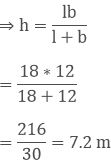Question: 9

Hameed has built a cubical water tank with lid for his house, with each other edge 1.5 m long. He gets the outer surface of the tank excluding the base, covered with square tiles of side 25 cm. Find how much he would spend for the tiles if the cost of tiles is Rs 360 per dozen.

Solution:

Given that

Hameed is getting 5 outer faces of the tank covered with tiles, he would need to know the surface area of the tank, to decide on the quantity of tiles required.

Edge of the cubical tank (a) = 1.5 m = 150 cm

So, surface area of the tank = 5 ∗ 150 ∗ 150 cm2= 180

Cost of 1 dozen tiles, i.e., cost of 12 tiles = Rs 360

Therefore, cost of one tile = Rs. 360/12 = Rs.30

So, the cost of 180 tiles = 180∗30 = Rs.5400

Question: 10

Each edge of a cube is increased by 50%. Find the percentage increase in the surface area of the cube.

Solution:

Let 'a' be the edge of the cube

Therefore the surface area of the cube = 6a2

i.e., S1 = 6a2

We get a new edge after increasing the edge by 50%

The new edge = a + 50/100∗a

= 3/2∗a

Considering the new edge, the new surface area is = 6∗ (3/2a)2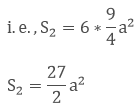Therefore, increase in the Surface Area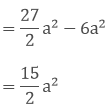So, increase in the surface area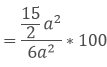= 15/12∗100

= 125%

Question: 11

The dimensions of a rectangular box are in the ratio of 2: 3: 4 and the difference between the cost of covering it with a sheet of paper at the rates of Rs 8 and Rs 9.50 per m2 is Rs 1248. Find the dimensions of the box.

Solution:

Let the ratio be ‘x’

Length (l) = 2x

Height (h) = 4x

Therefore, Total Surface area = 2[lb + bh + hl]

= 2(6x2 + 12x2 + 8x2)

= 52x2 m2

When the cost is at Rs.8 per m2

Therefore, the total cost of 52x2 = 8 ∗ 52x2

= Rs. 416x2

Taking the cost at Rs. 9.5 per m2,

Total cost of 52x2m2 = 9.5∗52x2

= Rs. 494x2

Therefore, the Difference in cost = Rs. 494x2 - Rs. 416x2

1248 = Rs. 78x2

x2 = 1248/78

x2 = 16

x = 4

Question: 12

A closed iron tank 12 m long, 9 m wide and 4 m deep is to be made. Determine the cost of iron sheet used at the rate of Rs 5 per meter sheet, a sheet being 2 m wide.

Solution:

Length (l) = 12 m

Height (h) = 4 m

Total surface area of the tank = 2[lb + bh + hl]

= 2[12*9 + 9*4 + 12*4]

= 2[108 + 36 + 48]

= 384 m2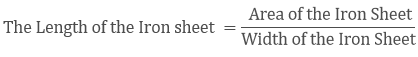= 384/2

= 192 m.

Cost of the Iron Sheet = Length of the Iron Sheet * Cost rate

= 192 * 5

= Rs. 960

Question: 13

Ravish wanted to make a temporary shelter for his car by making a box-like structure with the tarpaulin that covers all the four sides and the top of the car ( with the front face of a flap which can be rolled up). Assuming that the stitching margins are very small, and therefore negligible, how many tarpaulins would be required to make the shelter of height 2.5 m with base dimensions 4 m × 3m?

Solution:

Given That,

Shelter length = 4 m

Height = 2.5 m

The tarpaulin will be required for top and four sides of the shelter.

The Area of tarpaulin required = 2h(l + b) + lb

⇒ 2∗2.5 (4 + 3) + 4∗3

⇒ 5(7) + 12

⇒ 47 m2

Question: 14

An open box is made of wood 3 cm thick. Its external length, breadth and height are 1.48 m, 1.16 m and 8.3 dm. Find the cost of painting the inner surface of Rs 50 per sq. metre.

Solution:

Given Data:

Outer Dimensions

Length = 148 cm

Height = 83 cm

Inner Dimensions

Length = 148 - (2 * 3) = 142 cm

Breadth = 116 - (2 * 3) = 110 cm

Height = 83 - 3 = 80

Surface Area of the Inner region = 2h(l + b) + lb

= 2 * 80(142 + 110) + 142 * 110

= 2 * 80 * 252 + 142 * 110

= 55940 cm2

= 5.2904 m2

Hence, the cost of Painting the Surface Area of the Inner region= 5.2904* 50

= Rs. 279.70

Question: 15

The cost of preparing the walls of a room 12 m long at the rate of Rs 1.35 per square meter is Rs 340.20 and the cost of matting the floor at 85 paise per square meter is Rs 91.80. Find the height of the room.

Solution:

Given that,

Length of the room = 12m

Let the height of the room be 'h'

Area of 4 walls = 2(l + b)*h

According to the question

2(l + b) * h * 1.35 = 340.20

2(12 + b0 * h * 1.35 = 340.20Also Area of the Floor = l * b

Therefore, l ∗ b ∗ 0.85 = 91.80

⇒ 12 ∗ b ∗ 0.85 = 91.80

⇒ b = 9 m ... (2)

Substituting b = 9m in equation (1)

(12 + 9) * h = 126; h = 6 m

Question: 16

The dimensions of a room are 12.5 m by 9 m by 7 m. There are 2 doors and 4 windows in the room; each door measures 2.5 m by 1.2 m and each window 1.5 m by 1 m. Find the cost of painting the walls at Rs 3.50 per square meter.

Solution:

Given Length of the room = 12.5 m

Breadth of the room = 9 m

Height of the room = 7 m

Therefore, total surface area of the four walls = 2(l + b) * h

= 2(12.5 + 9) * 7

= 301 m2

Area of 2 doors = 2(2.5 *1.2)

= 6 m2

Area of 4 windows = 4 (1.5*1)

= 6 m2

Area to be painted on 4 walls = 301 - (6 + 6)

= 301 - 12

= 289 m2

Therefore, Cost of painting = 289 * 3.50

= Rs. 1011.5

Question: 17

The length and breadth of a hall are in the ratio 4: 3 and its height is 5.5 meters. The cost of decorating its walls (including doors and windows) at Rs 6.60 per square meter is Rs 5082. Find the length and breadth of the room.

Solution:

Let the length be 4a and breadth be 3a

Height = 5.5m [Given]

As mentioned in the question, the cost of decorating 4 walls at the rate of Rs. 6.60 per m2 is Rs. 5082.

Area of four walls * rate = Total cost of Painting

2(l + b) * h * 6.6 = 5082

2(4a + 3a) * 5.5 * 6.6 = 50827a = 70

a = 70/7

a = 10

Length = 4a = 4*10 = 40 m

Breadth = 3a = 3*10 = 30 m

Question: 18

A wooden bookshelf has external dimensions as follows: Height = 110 cm, Depth = 25 cm, Breadth = 85cm (See figure 18.5). The thickness of the plank is 5cm everywhere. The external faces are to be polished and the inner faces are to be painted. If the rate of polishing is 20 paise per cm2. Find the total expenses required for polishing and painting the surface of the bookshelf.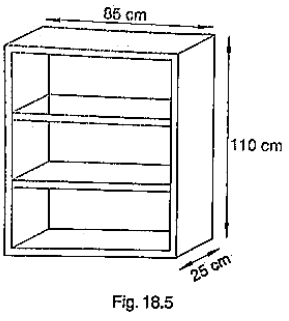Solution:

External length of book shelf = 85 cm

Height = 110 cm

External surface area of shelf while leaving front face of shelf

lh + 2(lb + bh)

[85 * 110 + 2(85 * 25 + 25 * 110)]

19100 cm2

Area of Front face = [85 * 110 - 75 * 100 + 2(75 * 5)] cm2

= 1850 + 750 cm2

= 2600 cm2

Area to be polished = 19100 + 2600 cm2

= 21700 cm2

Cost of polishing 1cm2 area = Rs. 0.20

Cost of polishing 21700 cm2 area = 21700 * 0.20

= Rs. 4340

Now, Length (l), breadth (b), height (h) of each row of book shelf is 75 cm, 20 cm and 30 cmrespectively.

Area to be painted in 1 row = 2(l + h)b + lh

[2(75 + 30) * 20 + 75 * 30)] cm2

(4200 + 2250) cm2

6450 cm2

Area to be painted in 3 rows = 3 * 6450

= Rs. 19350 cm2

Cost of painting 1cm2 area = Rs. 0.10

Cost of painting 19350 cm2 area = 19350 * 0.10

= Rs.1935

Total expense required for polishing and painting the surface of the bookshelf = 4340 + 1935

= Rs.6275

Question: 19

The paint in a certain container is sufficient to paint on an area equal to 9.375 m2, How many bricks of dimension 22.5cm × 10cm × 7.5cm can be painted out of this container?

Solution:

The paint in the container can paint the area,

A = 9.375 m2

= 93750 cm2 [Since 1 m = 100 cm]

Dimensions of a single brick,

Length (l) = 22.5 cm

Height (h) = 7.5 cm

We need to find the number of bricks that can be painted.

Surface area of a brick

A' = 2 (lb + bh + hl)

= 2(22.5 * 10 + 10 * 7.5 + 7.5 * 22.5)

= 2(225 + 75 + 168.75) = 937.50 cm2

Number of bricks that can be painted = A/A′

= 93750/937.5 = 100

Hence 100 bricks can be painted out of the container.
```### Course Features

• 728 Video Lectures
• Revision Notes
• Previous Year Papers
• Mind Map
• Study Planner
• NCERT Solutions
• Discussion Forum
• Test paper with Video Solution